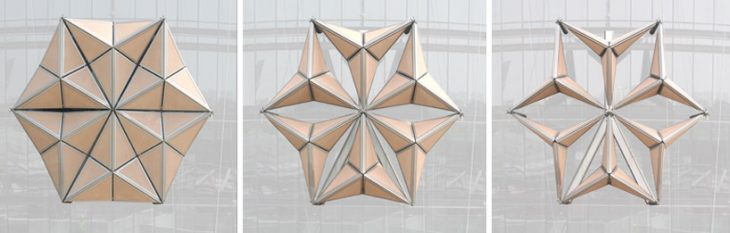# AL BAHAR TOWERSThe 145-meter towers’ shading system was developed by the computational design team at Aedas. The façade is a kinetic installation which answers to the amount of light; in this context, the sun, embracing the south and the east façades of the building,
The form is basically a repetition of hexagons, divided into triangles that creates the main components of the design. By sub-dividing these triangles through the middle points of its edges, moving parts of 3 are obtained for every triangle components. controlling the amount of sunlight that enters.## PSEUDO CODE

1. Draw a line in rhino and divide it n times.
2. Calculate the radius of a hexagon according to division number and the length of the curve.
3. Move the division points x times with the height of 1.5*radius.
4. Mask every other point to create a staggered pattern and place hexagons to these points.
5. Create a boundary surface from a hexagon.
6. Divide hexagons into triangles and divide these triangles from the center point into sub-triangles.
7. Extract the middle point of the outer edge of the triangle.
8. Rotate the center point along a circle around the center of sub-triangles.
9. Place a point in rhino to work as an attractor for the rotation angles.
10. Create lines using the rotating point and 2 other points.
11. Create a boundary surface by using the lines.
12. Merge all surfaces.